User’s Guide : Advanced Single Equation Analysis : Generalized Linear Models : Working with a GLM Equation

Working with a GLM Equation
EViews offers various views and procedures for a estimated GLM equation. Some, like the Gradient Summary or the coefficient Covariance Matrix view are self-explanatory. In this section, we offer relevant comment on the remaining views.
Residuals
The main equation output offers summary statistics for the sum-of-squared response residuals (“Sum squared resid”), and the sum-of-squared Pearson residuals (“Pearson SSR”).
The Actual, Fitted, Residual views and Residual Diagnostics allow you to examine properties of your residuals. The Actual, Fitted, Residual Table and Graph, show the fit of the unweighted data. As the name suggests, the Standardized Residual Graph displays the standardized (scaled Pearson) residuals.
The Residual Diagnostics show Histograms of the standardized residuals and Correlograms of the standardized residuals and the squared standardized residuals.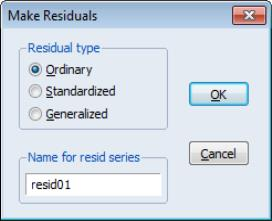The Make Residuals proc allows you to save the Ordinary (response), Standardized (scaled Pearson), or Generalized (score) residuals into the workfile. The latter may be useful for constructing test statistics (note, however, that in some cases, it may be more useful to compute the gradients of the model directly using Proc/Make Gradient Group).
Given standardized residuals SRES for equation EQ1, the unscaled Pearson residuals may be obtained using the command
series pearson = sres * @sqrt(eq1.@dispersion)
Forecasting
EViews offers built-in tools for producing in and out-of-sample forecasts (fits) from your GLM estimated equation. Simply click on the Forecast button on your estimated equation to bring up the forecast dialog, then enter the desired settings.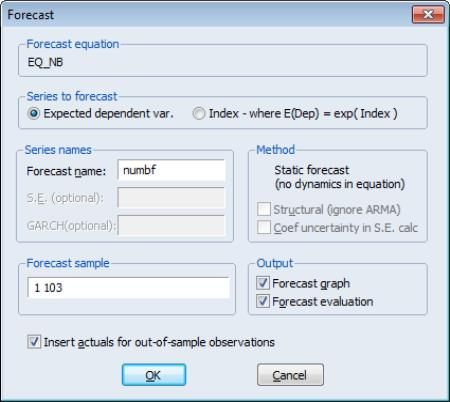You should first use the radio buttons to specify whether you wish to forecast the expected dependent variable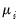or the linear index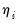.
Next, enter the name of the series to hold the forecast output, and set the forecast sample.
Lastly, specify whether you wish to produce a forecast graph and whether you wish to fill non-forecast values in the workfile with actual values or to fill them with NAs. For most cross-section applications, we recommend that you uncheck this box.
Click on OK to produce the forecast.
Note that while EViews does not presently offer a menu item for saving the fitted GLM variances or scaled variances, you can easily obtain results by saving the ordinary and standardized residuals and taking ratios ( “Residuals”). If ORESID are the ordinary and SRESID are the standardized residuals for equation EQ1, then the commands
series glmsvar = (oresid / sresid)^2
series glmvar = glmvar * eq1.@dispersion
produce the scaled variance and unscaled variances, respectively.
Lastly, you should use Proc/Make Model to create a model object for more complicated simulation from your GLM equation.
Testing
You may perform Wald tests of coefficient restrictions. Simply select View/Coefficient Diagnostics/Wald - Coefficient Restrictions, then enter your restrictions in the edit field. For the Papke-Wooldridge example above with Huber-White robust covariances, we may use a Wald test to evaluate the joint significance of AGE^2 and SOLE by entering the restriction “C(5)=C(6)=0” and clicking on OK to perform the test.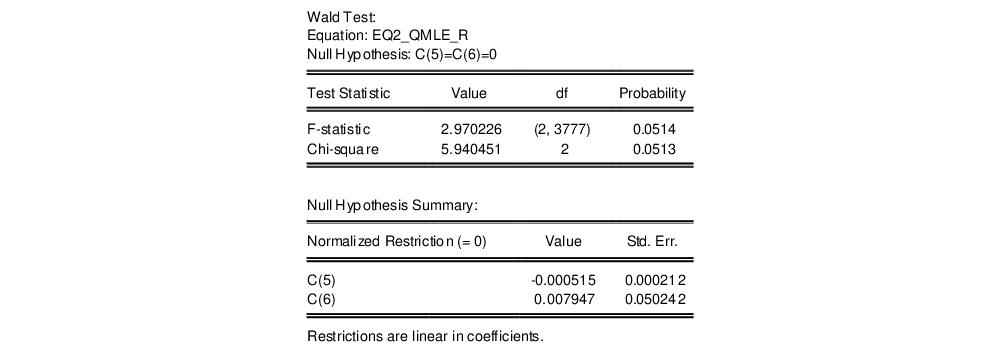The test results show joint-significance at just above the 5% level. The Confidence Intervals and Confidence Ellipses... views will also employ the robust covariance matrix estimates.
The Omitted Variables... and Redundant Variables... views and the Ramsey RESET Test... views are likelihood ratio based tests. Note that the RESET test is a special case of an omitted variables test where the omitted variables are powers of the fitted values from the original equation.
We illustrate these tests by performing the RESET test on the first Papke-Wooldridge QMLE equation with GLM covariances. Select View/Stability Diagnostics/Ramsey Reset Test... and change the default to include 2 fitted terms in the test equation.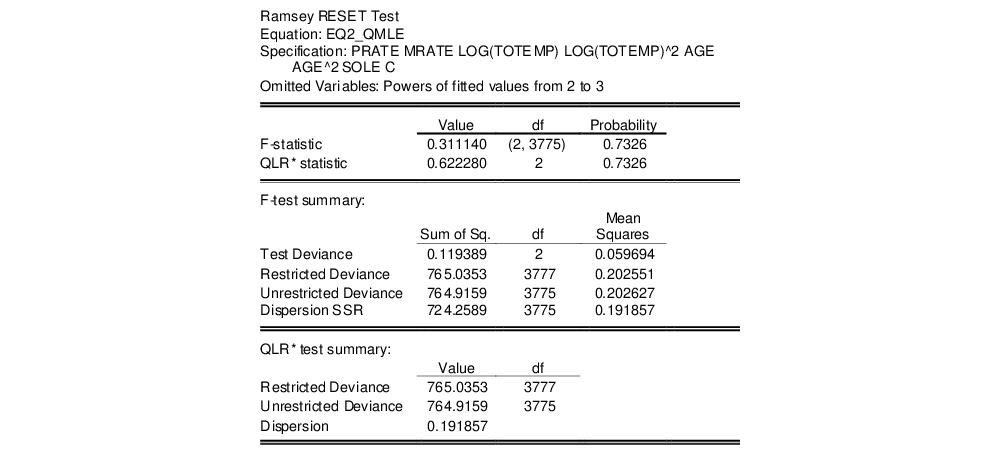The top portion of the output shows the test settings, and the test summaries. The bottom portion of the output shows the estimated test equation. The results show little evidence of nonlinearity.
Notice that in contrast to LR tests in most other equation views, the likelihood ratio test statistics in GLM equations are obtained from analysis of the deviances or quasi-deviances. Suppose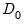is the unscaled deviance under the null and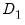is the corresponding statistic under the alternative hypothesis. The usual asymptotic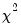likelihood ratio test statistic may be written in terms of the difference of deviances with common scaling,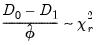(32.4)
as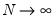, where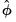is an estimate of the dispersion and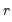is the fixed number of restrictions imposed by the null hypothesis.is either a specified fixed value or an estimate under the alternative hypothesis using the specified dispersion method. Whenandcontain the quasi-deviances, the resulting statistic is the quasi-likelihood ratio (QLR) statistic (Wooldridge, 1997).
If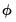is estimated, we may also employ the F-statistic variant of the test statistic: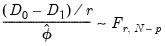(32.5)
where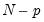is the degrees-of-freedom under the alternative andis an estimate of the dispersion. EViews will estimateunder the alternative hypothesis using the method specified in your equation.
We point out that the Ramsey test results (and all other GLM LR test statistics) presented here may be problematic since they rely on the GLM variance assumption, Papke and Wooldridge offer a robust LM formulation for the Ramsey RESET test. This test is not currently built-into EViews, but which may be constructed with some effort using auxiliary results provided by EViews (see Papke and Wooldridge, p. 625 for details on the test construction).